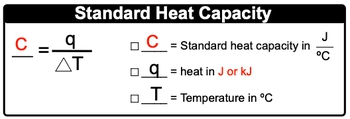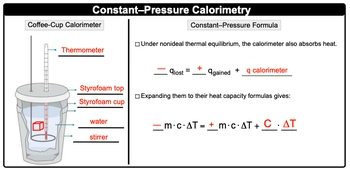Start typing, then use the up and down arrows to select an option from the list.## General Chemistry

Learn the toughest concepts covered in Chemistry with step-by-step video tutorials and practice problems by world-class tutors

8. Thermochemistry

# Constant-Pressure Calorimetry

Constant-Pressure Calorimetry uses a coffee cup calorimeter to determine the heat transfers occurring in a liquid solution.

Constant Pressure Calorimetry
1
concept

## Constant-Pressure Calorimetry1m
Play a video:
now a calorie meter is just a container, usually insulated against heat loss that contains a liquid with a given heat capacity, we're going to say, usually when a heated object is placed in a liquid, both the liquid and the container that it's in will absorb this released heat. And we're gonna say here that are standard he capacity, which is capital C for a calorie meter, if just the amount of heat required to change its temperature. Now, the weight of the calorie meters, usually unknowns. We're going to exclude it from our our Mass in terms of the formula now standard he capacity equals capital. C equals Q, which is our heat divided by changing temperature. So our standard he capacity is usually in units of jewels over degrees Celsius. Now this temperature could be given to us in Kelvin, and if it is given to us in Kelvin, then the temperature must be converted to Kelvin as well. The units here both have to match one another. Here are Q, which is our heat can be in jewels or in killing jewels, so always be on the lookout for that to make sure units all match so that they cancel out toe, isolate the missing variable2
example

## Constant-Pressure Calorimetry Example 11m
Play a video:
What is the standard heat capacity in kill jewels per degree? Celsius off a calorie motor that absorbs 87 jewels at its temperature as his temperature goes from 33 degrees Celsius to 38. degree Celsius. Alright, so your standard capacity A C capital C and remembered equals Q divided by changing temperature. Here, we need to convert our jewels into killer jewels because they want the unit and kill jewels. So we have 87.0 jewels. Remember that 1 kg is 10 to the three jewels here, so that's 30.87 killing jewels. Take that and plug it in. And then Delta teas are changing temperature final temperature minus initial temperature. So when we do that, we're going to get our answer in killer jewels over degrees Celsius. This comes out to be 0.171 killer jewels over degrees Celsius. Here are answers gonna be given to us in three Sig figs because I'm using 87.0 jewels as my reference here. Our temperatures could also be used. This has two significant figures. This has three. But just to give more detailed to our answer, we're gonna go with three significant figures
3
concept

## Constant-Pressure Calorimetry2m
Play a video:
now constant pressure Kalorama tree is associated with our coffee cup cal or emitter. Now we're going to say here, it uses the coffee cup cal or emitter to determine he transfers occurring in a liquid solution. Now a cup, coffee cup caliber mature is basically an insulated styrofoam cup with a lid and we're gonna say we call it constant pressure because the calorie meter measuring heat is open to the atmosphere where pressure is going to be fixed. So here we're gonna take a look at the images provided to us. We're gonna start out with our coffee cup calorie meter here on the left, when we look at this coffee cup calorie meter, we're gonna say it has some key portions to it. One of them being this thermometer given to us on top. So that's gonna measure my temperature difference. Then we're gonna have here are styrofoam cover or styrofoam top here. This portion just represents the styrofoam cup itself. In here we have our water and then finally here we have our stir when we place the heated object inside of here, we're gonna be able to measure the amount of heat that's being released. Now realize here when it comes to the constant cup on constant pressure cal or emitter and this coffee cup calorie meter. We have formulas that are associated with it. We're gonna say when both the liquid and calorie meter absorb heat from the hot object. We get the heat lost by the object. So minus q plot equals plus he gained by the water plus the heat also gained by the Calgary emitter. Now we can expand this a little bit further, we're gonna say expanding them to their heat capacity formulas where Q equals M. Cat, we're gonna have Q lost is minus M. Cat. Q. Gained by the water is positive and cat. And remember for the cow limiter, the mass of it is usually unknown. So we ignore its mass within our formula. So it just becomes the heat capacity of the cal or emitter, which is capital C. Times change in temperature. So that becomes our equation for constant pressure kalorama tree, it's going to be minus M. Cat equals plus M. Cat. Plus the specific will the heat capacity of the calorie meter times its change in temperature.4
example

## Constant-Pressure Calorimetry Example 24m
Play a video:
here, we're told when 60 g of lead at 68.3 degrees Celsius is poured into 90 g water at 30 degrees Celsius. Within a coffee cup calorie meter, the temperature increases to 48 degrees Celsius. Based on this information, what is the heat capacity of the calorie meter? We're told the specifically capacity specific heat of lead in water are 0.1 to 8 and 4.184 respectively. All right, so we're gonna plug. Well, first of all, we need to realize we have our container with water and we're placing it placing into it. Our lead lead is at a higher temperature than the water is. Remember, the hotter object always releases its heat, so it's gonna release its heat. So the water is releasing its heat. So negative Q of water? No negative. You bled? Sorry. The object that's releasing its hey, he equals positive Q of water, which is absorbing it, plus Q of the calorie meter. The calorie meter is absorbing some of it as well because they tell us that we need to find a T capacity. We don't need to find a T capacity. If it was involved with in the calculation. All right, so if their cues are involved, that means they're m. Katz for some of them are involved. So negative m cat of our lead equals positive and cat of the water Remember calorie emitters. Their masses tend to be unknown, so it's just the heat capacity that they have times the change in temperature. Alright, so here we're gonna say negative 60 g of our lead times. It's specific heat capacity, times the change in temperature. We're told that the temperature increases to 48 degrees Celsius that the final temperature for everyone. So here the change in temperature for the lead is the final temperature, minus its initial temperature of 68.3 degrees Celsius. That equals positive and cat of water and cat um so water is 90 g of water times. It's specific heat capacity times its final temperature minus the initial temperature that it had. Plus, we don't know the heat capacity of the Cal. Where matter? That's what we're looking for. And here's the thing. Before we add the lead to the water. It was just the water in the calorie Mitter hanging out with each other so they both must have been at the same initial temperature, so change in temperature would be 48 degrees Celsius minus the initial temperature of the calorie meter, which again it was with water and we assume they both were at the same initial temperature of 30 degrees Celsius. All we have to do now solve for this one missing variable. So what we're gonna do now is we're going to multiply everything here on this side. Grams will cancel out. Degrees Celsius will cancel out, will have jewels left on this side, which comes out to 1. 55.904 jewels equals grams. Cancel out degrees Celsius, cancel out. So we're gonna have everything here also in jewels. So that is 6778.8 jewels. Plus, when you subtract these, that's gonna give me 18 degrees Celsius times my specific capacity or my heat capacity of C gotta We gotta isolate our heat capacity of C. So next we're going to subtract this from both sides here. So when we do that, we're gonna have now negative 66. actually, negative. 66 to 2 point 176 equals 18 degrees Celsius. Times my heat capacity of seat divide out 0 degrees Celsius. Remember Jules air still here? So 18.0 degree Celsius heat capacity is a number that is positive, So this is an absolute terms. When we do that, that's jewels divided by degrees Celsius. So here my heat capacity, which is capital C, equals 3 67. jewels over degrees Celsius. So that would be the heat capacity of our calorie emitter within this given question.
5
Problem

A 115.6 g piece of copper metal at 182.5 ºC is placed into 120.0 mL of methylene chloride at 31.0 ºC within a coffee-cup calorimeter. If the final temperature of the solution is 50.3 ºC, what is the specific heat of methylene chloride? Assume the calorimeter absorbs a negligible amount of heat. The specific heat of copper is 4.184 J/g ∙ ºC and the density of methylene chloride is 1.33 g/cm3.

6
Problem

You place 75.0 mL of 0.100 M NaOH in a calorimeter at 25.00 ºC and carefully add 55.0 mL of 0.200 M HNO3, also at 25.00 ºC. After stirring, the final temperature is 53.35 ºC. Calculate the enthalpy ∆Hrxn (in J/mol) for the formation of water. (Specific heat capacity, Cs, and density of the solution:4.184 J/g∙K and 1.00 g/mL).